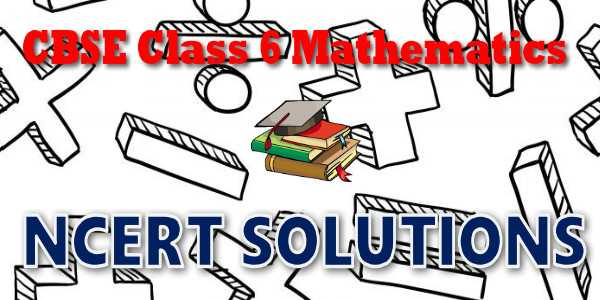No products in the cart.

# CBSE - Class 06 - Mathematics - Whole Numbers - NCERT Solutions## CBSE, JEE, NEET, NDA

Question Bank, Mock Tests, Exam Papers

NCERT Solutions, Sample Papers, Notes, Videos

## NCERT Solutions for Mathematics Whole Numbers## myCBSEguide App

Complete Guide for CBSE Students

NCERT Solutions, NCERT Exemplars, Revison Notes, Free Videos, CBSE Papers, MCQ Tests & more.

# NCERT solutions for class 6 Mathematics Whole Numbers## NCERT Class 6 Mathematics Chapter-wise Solutions

Maths Part I

• Chapter 1 - Knowing Our Numbers
• Chapter 2 - Whole Numbers
• Chapter 3 - Playing with Numbers
• Chapter 4 - Basic Geometrical Ideas
• Chapter 5 - Understanding Elementary Shapes
• Chapter 6 - Integers
• Chapter 7 - Fractions
• Chapter 8 - Decimals
• Chapter 9 - Data Handling
• Chapter 10 - Mensuration
• Chapter 11 - Algebra
• Chapter 12 - Ratio and Proportion
• Chapter 13 - Symmetry
• Chapter 14 - Practical Geometry

## CBSE class 6th Mathematics have one books. Book has chapters and topics.

• NCERT Mathematics Book Class 6

Here is the list of topics covered under each chapter of class 6 Mathematics NCERT text book.

• Exercise 1.1
• Exercise 1.2
• Exercise 1.3

• Exercise 2.1
• Exercise 2.2
• Exercise 2.3

• Exercise 3.1
• Exercise 3.2
• Exercise 3.3
• Exercise 3.4
• Exercise 3.5
• Exercise 3.6
• Exercise 3.7

• Exercise 4.1
• Exercise 4.2
• Exercise 4.3
• Exercise 4.4
• Exercise 4.5
• Exercise 4.6

• Exercise 5.1
• Exercise 5.2
• Exercise 5.3
• Exercise 5.4
• Exercise 5.5
• Exercise 5.6
• Exercise 5.7
• Exercise 5.8
• Exercise 5.9

• Exercise 6.1
• Exercise 6.2
• Exercise 6.3

• Exercise 7.1
• Exercise 7.2
• Exercise 7.3
• Exercise 7.4
• Exercise 7.5
• Exercise 7.6

• Exercise 8.1
• Exercise 8.2
• Exercise 8.3
• Exercise 8.4
• Exercise 8.5
• Exercise 8.6

• Exercise 9.1
• Exercise 9.2
• Exercise 9.3
• Exercise 9.4

### 10. Mensuration

• Exercise 10.1
• Exercise 10.2
• Exercise 10.3

### 11. Algebra

• Exercise 11.1
• Exercise 11.2
• Exercise 11.3
• Exercise 11.4
• Exercise 11.5

### 12. Ratio and Proportion

• Exercise 12.1
• Exercise 12.2
• Exercise 12.3

### 13. Symmetry

• Exercise 13.1
• Exercise 13.2
• Exercise 13.3

### 14. Practical Geometry

• Exercise 14.1
• Exercise 14.2
• Exercise 14.3
• Exercise 14.4
• Exercise 14.5
• Exercise 14.6

## NCERT Solutions Chapter 2: Whole Numbers

As we know, we use 1, 2, 3, 4,... when we begin to count. They come naturally when we start counting. Hence, mathematicians call the counting numbers as Natural numbers. Predecessor and successor Given any natural number, you can add 1 to that number and get the next number i.e. you get its successor. The successor of 16 is 16 + 1 = 17, that of 19 is 19 +1 = 20 and so on. The number 16 comes before 17, we say that the predecessor of 17 is 17–1=16, the predecessor of 20 is 20 – 1 = 19, and so on. The number 3 has a predecessor and a successor. What about 2? The successor is 3 and the predecessor is 1. Does 1 have both a successor and a predecessor? We can count the number of children in our school; we can also count the number of people in a city; we can count the number of people in India. The number of people in the whole world can also be counted. We may not be able to count the number of stars in the sky or the number of hair on our heads but if we are able, there would be a number for them also. We can then add one more to such a number and get a larger number. In that case we can even write the number of hair on two heads taken together. It is now perhaps obvious that there is no largest number. Apart from these questions shared above, there are many others that can come to our mind when we work with natural numbers. You can think of a few such questions and discuss them with your friends. You may not clearly know the answers to many of them !

## NCERT Solutions for Class 6th Mathematics

NCERT Solutions Class 6 Mathematics PDF (Download) Free from myCBSEguide app and myCBSEguide website. Ncert solution class 6 Mathematics includes text book solutions from  NCERT Solutions for CBSE Class 6 Mathematics have total 14 chapters. Class 6 Mathematics ncert Solutions in pdf for free Download are given in this website. Ncert Mathematics class 6 solutions PDF and Mathematics ncert class 6 PDF solutions with latest modifications and as per the latest CBSE syllabus are only available in myCBSEguide.

## NCERT Solutions for Class 5

NCERT Solutions for Class 4

## NCERT Solutions for Class 3## myCBSEguide

Trusted by 1 Crore+ Students

#### Question Paper Creator

• Create papers in minutes
• Print with your name & Logo